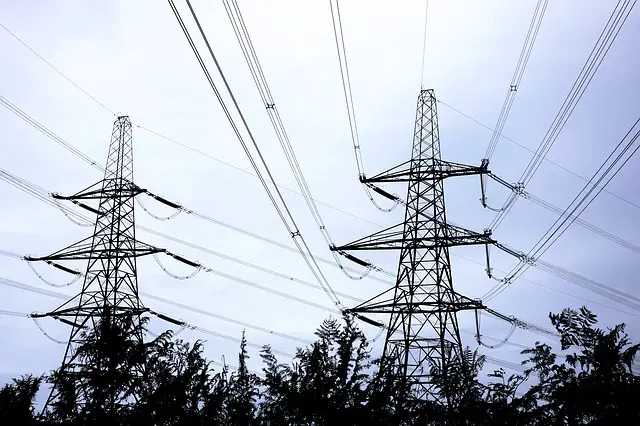# Poisson’s ratio, Strain energy & Thermal Stress – expressions

Last updated on October 3rd, 2021 at 09:37 am

Today we will briefly discuss a couple of very important terms and concepts from the elasticity chapter. Those are Poisson’s ratio, Strain energy, Thermal Stress, etc. We will also derive expressions for these.

This post is the 3rd post of the series. Already in our last 2 posts, we have discussed the meaning of Elasticity and related concepts like Stress, Strain, Hooke’s Law, and modulus of elasticity.

## Poisson’s Ratio formula

When a wire is stretched, its length increases but its diameter is reduced.
In other words, both shape and volume change under Longitudinal Stress.

Here comes Poisson’s ratio to measure 2 resulting strains because of this longitudinal stress. These 2 strains are known as Lateral Strain and Longitudinal Strain.

Lateral Strain = (change in diameter) / (Original diameter) = ΔD/D

Longitudinal Strain = (change in length) / (original length) = ΔL/L

Now, the Poisson’s ratio (σ) is derived from the ratio of lateral strain and longitudinal strain.

Poisson’s ratio σ = Lateral Strain / Longitudinal Strain = (ΔD/D)/(ΔL/L)
σ = (ΔD . L ) / ( D. ΔL) ……………. (1)

Poisson’s ratio is dimensionless and unitless as well.

### Strain energy

Strain energy definition: We can define Strain Energy as the energy stored in a strained wire because of longitudinal stress.

Say,  F is the force applied on the cross-sectional surface of area A. This causes a length change of ΔL for a wire of original length L.

So work done on the wire = Energy stored in the wire = Average force.Displacement = (F/2) . ΔL

Now, let’s find out the expression of the energy stored per unit volume of the wire.
The volume of the wire = A L

Energy stored per unit volume =  (1/2) (F . ΔL )/(A . L)
= (1/2) (F/A) (ΔL/L)

Strain Energy stored per unit volume in a strained wire = (1/2). (Stress). (Strain) ……….(2)

### Thermal Stress

As temperature changes, it affects the length of a solid wire, and eventually causes Thermal stress.

Let’s find out the expression of Thermal Stress.

Say, F is the applied force on a surface with area A.

If the initial length is L and α be the coefficient of linear expansion, then the change in length ΔL due to Δt is as follows.
ΔL = α L Δt …………….(3)

Young Modulus = Y = (FL)/(ΔL.A) …… (4)

From (3) and (4)

So F =( Y ΔL.A )/L

= (Y α L Δt .A )/L = Y α Δt .A
F = Y α Δt .A …………… (5)

So, Thermal Stress = F/A

Thermal Stress = Y α Δt .A/A= Y α Δt …….(6)

## Formulas derived

1 ]

Poisson’s ratio σ = Lateral Strain / Longitudinal Strain = (ΔD/D)/(ΔL/L)
σ = (ΔD . L ) / ( D. ΔL)

2 ]

Strain Energy stored per unit volume = (1/2). (Stress). (Strain)

3 ]

Thermal Stress = Y α Δt .A/A= Y α Δt

## Related posts

Dear Readers, here we have discussed Poisson’s ratio, Strain energy, Thermal Stress, and derived expressions for these. Here is a list of related posts for your study.

Elasticity

Hooke’s law